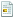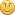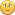November 27, 2021, 04:47:15 pm

## ESP32S2 devkit lipo battery voltage measurement

Started by xtrinch, May 08, 2021, 10:14:32 pm

#### xtrinch

#####May 08, 2021, 10:14:32 pm
Hello,

I've got a question about the BAT_SENS pin on GPIO8.
So I've done some measurements on the pin and this is how I calculated my conversion:

double voltagePerNum = 3.3/8192.0; // 3.3V resolution, 13 bit ADC
double vBat = (vBatMeasured * (470000+4700000)) / 470000.0; // use the voltage divider equation to calculate the input battery voltage

I took the resistor values from the datasheet.

When a LiPo battery with an actual voltage of 4.17V is connected, the ADC value is 1096 which makes the calculated value 4.85V.

My question is whether the values are expected to be off by that much and I should just multiply with a calibration factor (which in my case is 0.85 * calculated voltage)? I couldn't find in the BOM what the accuracy of the resistors used in the voltage divider are. Is there something else I can tune to make the measurement more accurate (provided that my calculation is in fact correct at all)?

Any help would be greatly appreciated.

#### findmyname

#####May 16, 2021, 07:04:28 pm #1
I'm trying to figure-out the same thing.
Any reply would be appreciated#### LubOlimex

#####May 18, 2021, 10:31:54 am #2
I think the reference is not 3.3V, but between 0 and 1.1-1.2V:

Also ADC has to be calibrated before using it, to find true max.

findmyname did you solder together jumper BAT_SENS_E1 first?
Technical support and documentation manager at Olimex

#### findmyname

#####May 18, 2021, 11:55:36 am #3
Quote from: LubOlimex on May 18, 2021, 10:31:54 amfindmyname did you solder together jumper BAT_SENS_E1 first?

Yeah I did, I also read some value from it but it seems to be out of range.

Quote from: LubOlimex on May 18, 2021, 10:31:54 amI think the reference is not 3.3V, but between 0 and 1.1-1.2V:
I tried to multiple it by 3.3 but still not ideal but I'm not really sure how should looks exact equation.

Thx

#### LubOlimex

#####May 18, 2021, 12:39:03 pm #4 Last Edit: May 18, 2021, 12:41:11 pm by LubOlimex
QuoteI tried to multiple it by 3.3 but still not ideal but I'm not really sure how should looks exact equation.

Thx

I meant 3.3V seems straight wrong. Use 1.1 instead of 3.3.

double voltagePerNum = 1.1/8192.0;

Also it is not clear if it is exactly 1.1, so you first need to do ADC calibration as pointed here to get the exact max value:

Technical support and documentation manager at Olimex

#### xtrinch

#####May 20, 2021, 12:36:28 pm #5 Last Edit: May 20, 2021, 12:55:08 pm by xtrinch
I read that part about the reference being 1.1, but the calculated values were so off, if I used that, that I discarded it.

Here's an example, using the following code:
double voltagePerNum = 1.1/8192.0; // 1.1V resolution, 13 bit ADC
double vBat = (vBatMeasured * (470000+4700000)) / 470000.0; // use the voltage divider equation to calculate the input battery voltage

The analog value read is as mentioned in the original post 1096, corresponding to multimeter measured voltage of 4.17V, which gives us result:
(((1.1/8192.0)*1096)*(470000+4700000))/470000.0 = 1.62 V
The ADC calibration wouldn't have made any difference here (but thanks I will definitely do that, although I've read the ESP32S2 comes factory calibrated already), the values would be greatly off.

#### xtrinch

#####June 06, 2021, 11:06:15 pm #6 Last Edit: June 07, 2021, 03:44:20 pm by xtrinch
Okay so I dug in a bit and found out more.

Default attenuation is set to 11db, which makes the range around 2.6V. However, since the voltage divider of this board is set so that our input voltage is much lower than 1V, we can use zero attenuation (that makes the range around 1.1V).

For arduino, we can do that with:

So then this code (works only with attenuation 0db which is not the default):

double voltagePerNum = 1.1/8192.0; // <-- 1.1=vRef
double vBat = (vBatMeasured * (470000+4700000)) / 470000.0;

Gives the same result as:

double vBat = (analogReadMilliVolts(BAT_SENS_PIN) * (470000+4700000)) / 470000.0;

If you use the analogReadMilliVolts you do not need to care about the set attenuation as it will convert it into the correct value for you depending on the attenuation set.

I then routed my VRef to a GPIO with the following code:

if (status == ESP_OK) {
printf("v_ref routed to GPIO\n");
} else {
printf("failed to route v_ref\n");
}

Measured with a multimeter, my actual vRef is 1.168. Factory calibration value is 1.1. Little bit of a difference.

An example calculation with ADC value 2937 then gives:

(((1.168/8192.0)*2937)*(470000+4700000))/470000.0 = 4.60 V

Measured battery voltage for that ADC value was however 3.8V.

Extremely inaccurate, it seems? Off by 0.8V. The voltage divider is set to 0.09 of the original voltage, making any error 11 times multiplied. My error in measurement is 0.07 V (reeally not that much), multiplied by 11, gives us the 0.8V difference. I wish you guys had set the voltage divider to allow for 2.6 max voltage (maximum that the ADC with maximum attenuation can comfortably handle). That would make the error multiplied by 1.something, instead of 11.

Something like two times 2.5M resistors:
2500000/(2500000 + 2500000) * 4.2 = 2.1 max voltage on pin

It seems that the USB and regular devkit LiPo boards have a different choice of resistors, the regular LiPo has two times 470k resistors - how come that was abandoned? Could have just made both of them bigger if the 4uA extra power consumption was too much?

#### findmyname

#####September 03, 2021, 04:30:45 pm #7
@xtrinch very nice analysisOtherwise the board will just stop working when deployed in the field